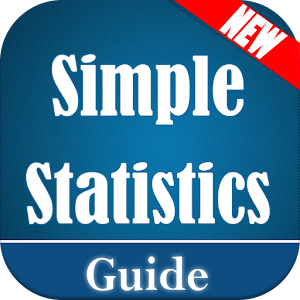## Simple Statistics - Lumos Educational App Store41
Price -NA
\$A

#### DESCRIPTION:

Statistics is a branch of mathematics dealing with the collection, analysis, interpretation, presentation, and organization of data. In applying statistics to, e.g., a scientific, industrial, or social problem, it is conventional to begin with a statistical population or a statistical model process to be studied. Simple Statistics application cover following topics of Statistics 0. Adjusted R-Squared 1. Analysis of Variance 2. Arithmetic Mean 3. Arithmetic Median 4. Arithmetic Mode 5. Arithmetic Range 6. Bar Graph 7. Best Point Estimation 8. Beta Distribution 9. Binomial Distribut

#### OVERVIEW:

Simple Statistics is a free educational mobile app By Mobile Coach.It helps students in grades HS practice the following standards HSS.ID.A.1,HSS.ID.A.2,HSS.ID.A.3.

This page not only allows students and teachers download Simple Statistics but also find engaging Sample Questions, Videos, Pins, Worksheets, Books related to the following topics.

1. HSS.ID.A.1 : Represent data with plots on the real number line (dot plots, histograms, and box plots)..

2. HSS.ID.A.2 : Use statistics appropriate to the shape of the data distribution to compare center (median, mean) and spread (interquartile range, standard deviation) of two or more different data sets..

3. HSS.ID.A.3 : Interpret differences in shape, center, and spread in the context of the data sets, accounting for possible effects of extreme data points (outliers)..

HS

#### STANDARDS:

HSS.ID.A.1
HSS.ID.A.2
HSS.ID.A.3
HSS.ID.A.4
HSS.ID.B.5
HSS.ID.B.6
HSS.ID.C.7
HSS.ID.C.8
HSS.ID.C.9

Developer: Mobile Coach

Software Version: 2.1.2

Category: Education

### RELATED APPSEdSearch WebSearch